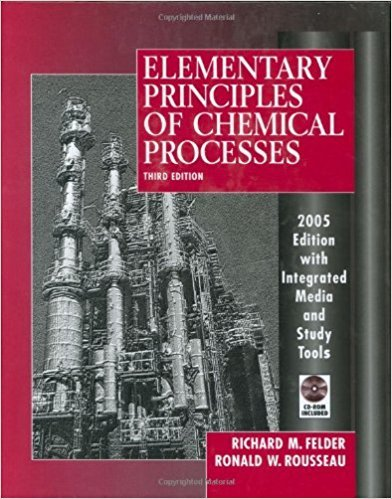×
Get Full Access to Elementary Principles Of Chemical Processes - 3 Edition - Chapter 2 - Problem 2.25
Get Full Access to Elementary Principles Of Chemical Processes - 3 Edition - Chapter 2 - Problem 2.25

×

# A seed crystal of diameter D (mm) is placed in a solutionISBN: 9780471687573 143

## Solution for problem 2.25 Chapter 2

Elementary Principles of Chemical Processes | 3rd Edition

• Textbook Solutions
• 2901 Step-by-step solutions solved by professors and subject experts
• Get 24/7 help from StudySoup virtual teaching assistantsElementary Principles of Chemical Processes | 3rd Edition

4 5 1 370 Reviews
10
0
Problem 2.25

A seed crystal of diameter D (mm) is placed in a solution of dissolved salt, and new crystals are observed to nucleate (form) at a constant rate r (crystals/min). Experiments with seed crystals of different sizes show that the rate of nucleation varies with the seed crystal diameter as r(crystals/min) = 200D - lOD2 (D in mm) (a) What are the units of the constants 200 and 10? (Assume the given equation is valid and therefore dimensionally homogeneous.) (b) Calculate the crystal nucleation rate in crystals/s corresponding to a crystal diameter of 0.050 inch. (c) Derive a formula for r(crystals/s) in terms of D(inches). (See Example 2.6-1.) Check the formula using the result of part (b).

Step-by-Step Solution:

Problem 2.25A seed crystal of diameter D (mm) is placed in a solution of dissolved salt, and new crystals areobserved to nucleate (form) at a constant rate r (crystals/min). Experiments with seed crystals ofdifferent sizes show that the rate of nucleation varies with the seed crystal diameter asr(crystals/min) = 200D - 10D2 (D in mm)(a) What are the units of the constants 200 and 10 (Assume the given equation is valid andtherefore dimensionally homogeneous.)(b) Calculate the crystal nucleation rate in crystals/s corresponding to a crystal diameter of0.050 inch.(c) Derive a formula for r(crystals/s) in terms of D(inches). (See Example 2.6-1.) Check theformula using the result of part (b). Step-by-step solution Step 1 of 5 ^a)The relation between rate of nucleation and seed crystal diameter is given below: r( crysta)s= 200D 10D (D in mm) minThe first step is to examine which units are on which side. Since both sides must match, we candetermine what is missing in the variables. crystals 2 r( min ) = 200D × D (mm) 10 × D (mm) ( cminta)s= (mm) × (mm) × We must multiply the two terms in the right side of equation by units that will result inystals ( min ) crystals crystals 2 crystals ( min ) = (mm) × ( mm . min (mm ) × ( mm . minAbove we see that the units must cancel out to be crystals/min, therefore the units for the first crystals crystalsconstant and second constant are (mm . min and ( mm . min respectively.

Step 2 of 5

Step 3 of 5

##### ISBN: 9780471687573

This full solution covers the following key subjects: crystals, crystal, rate, diameter, seed. This expansive textbook survival guide covers 13 chapters, and 710 solutions. The full step-by-step solution to problem: 2.25 from chapter: 2 was answered by , our top Chemistry solution expert on 11/15/17, 02:42PM. This textbook survival guide was created for the textbook: Elementary Principles of Chemical Processes, edition: 3. Elementary Principles of Chemical Processes was written by and is associated to the ISBN: 9780471687573. The answer to “A seed crystal of diameter D (mm) is placed in a solution of dissolved salt, and new crystals are observed to nucleate (form) at a constant rate r (crystals/min). Experiments with seed crystals of different sizes show that the rate of nucleation varies with the seed crystal diameter as r(crystals/min) = 200D - lOD2 (D in mm) (a) What are the units of the constants 200 and 10? (Assume the given equation is valid and therefore dimensionally homogeneous.) (b) Calculate the crystal nucleation rate in crystals/s corresponding to a crystal diameter of 0.050 inch. (c) Derive a formula for r(crystals/s) in terms of D(inches). (See Example 2.6-1.) Check the formula using the result of part (b).” is broken down into a number of easy to follow steps, and 116 words. Since the solution to 2.25 from 2 chapter was answered, more than 809 students have viewed the full step-by-step answer.

Unlock Textbook Solution